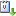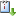Usted está aquí: H-Cycles and H-Paths in H-Edge Coloured Digraphs

# H-Cycles and H-Paths in H-Edge Coloured Digraphs

Ingrid Torres (IMUNAM)
Ponente:
Cuándo 24/08/2011 de 18:00 a 19:00 Sala de café, Instituto de Matemáticas Ingrid Chantal Torres RamosvCaliCal

A classical problem in Digraph Theory is find sufficient conditions for the existence of a kernel in a digraph, since it is a property that does not have all digraph. Moreover, Chvátal showed that deciding if a graph possesses a kernel is an NP-complete problem. The concept of kernel was introduced by Von Neumann and Morgenstern in the area of Games Theory.

A set N of V(D) is said to be an H-kernel by paths in D if it satisfies the following two conditions: 1) for every two different vertices u, v in N does not exist an H-path between them and; 2) for every vertex x in V(D)-N exists a vertex y in N such that there is an H-path in D from x to y.

In this talk I will introduce the concept of H-separation of an digraph and we have proved that if H is a strongly transitive digraph and D is a digraph H-colored, P={D₁,D₂} an H-separation of D such that:

1. Every cycle of D that is contained in Dᵢ is an H-cycle for i=1,2.

2. D does not contain a (D₁,D,D₂) H-subdivision of C₃.

3. If (u,z,w,x₀) is a (D₁,D,D₂) H-subdivision of P₃ then there exist some of the following H-paths: an H-path from u to x₀ or an H-path from x₀ to u.

Then D has a H-kernel by paths.

Seminario de Becarios